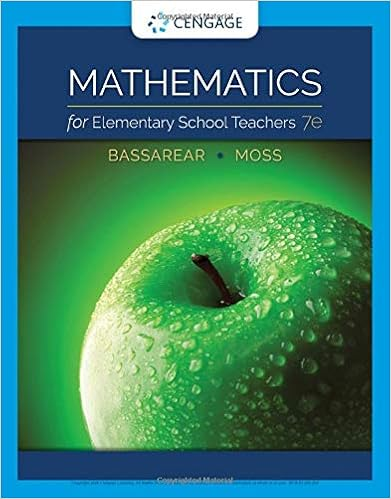homework 3 - Math 1313 Homework 3 Section 2.1 Answer...

• Homework Help
• 2
• 50% (4) 2 out of 4 people found this document helpful

This preview shows page 1 - 2 out of 2 pages.

We have textbook solutions for you!
The document you are viewing contains questions related to this textbook.The document you are viewing contains questions related to this textbook.
Chapter 10 / Exercise 6
Mathematics for Elementary School Teachers
BassarearExpert Verified
Math 1313 Homework 3 Section 2.1 Answer questions 1 - 3 for problem number 10 from the book . 1. Give the corners of the feasible set. a. (0,0), (0,5), (5,0) b. (0,0), (0,5), (3,0) c. (0,0), (0,5), (3,0), (2,3) d. (3,0), (5,0), (2,3) e. (0,5), (0,9), (2,3)
1
2. Give the maximum value(part b).
3. Give the minimal value(part c)
Answer questions 4 - 6 for problem number 12 from the book . 4. Give the corners of the feasible set.
5. Give the maximum value. a. 0 b. 80 c. 160 d. 28 e. 42
6. Give the minimum value.
We have textbook solutions for you!
The document you are viewing contains questions related to this textbook.The document you are viewing contains questions related to this textbook.
Chapter 10 / Exercise 6
Mathematics for Elementary School Teachers
BassarearExpert Verified
Math 1313 Homework 3 Section 2.1 2 Use the following problem to answer questions 7 and 8.
•••